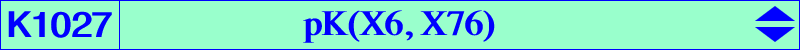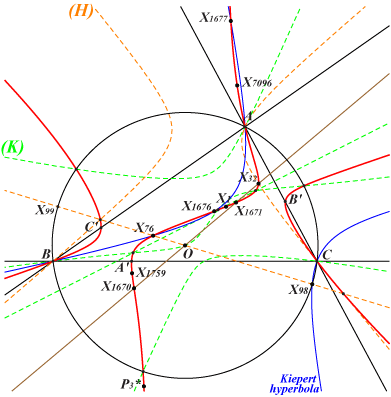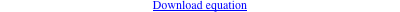∑ a^4 y z (b^2 y - c^2 z) = 0 ∑ a^2 x^2 (c^4 y - b^4 z) = 0 ∑ b^2 c^2 x (c^2 y^2 - b^2 z^2) = 0X(1), X(32), X(76), X(1670), X(1671), X(1676), X(1677), X(1759), X(7096) excenters vertices of the cevian triangle of X(76) P3 = X(76)-Ceva conjugate of X(32) = tertiary pivot = tangential of X(32) P3*, its isogonal conjugate, the tangential of X(1759) other points below Geometric properties :K1027 is a very simple isogonal pK introduced in the study of Q142. It is also mentioned in the page K704 as a member of the pencil of pK(X6, P) with P on the line X(32), X(76), X(384), X(385), X(736), X(2233), X(3114), etc, containing K020, K128. The orthic line of K1027 is the line X(3), X(76), X(98), X(99), etc, meeting K1027 at X(76) and two other (not always real) points on the rectangular circum-hyperbola (H) that passes through X(32). K1027 meets the circumcircle at the same points as (K) = pK(X1176, X83). The three other common points of these two cubics lie on the line X(2), X(6). K1027 is anharmonically equivalent to pK(X1501, X2), pK(X3117, X2), pK(X2, X561), pK(X32, X75), pK(X3094, X75) and any pK(P, P) with P on the line with equation ∑ b^6 c^6 (y - z) = 0. *** A related Cundy-Parry transformation As in CL037, two transformations 𝝓 and 𝝍 are defined as follows : 𝝓(M) = MX(76) /\ M*X(32) and 𝝍(M) = MX(32) /\ M*X(76), where M* is the isogonal conjugate of M. The properties in CL037 are easily adapted, in particular : • 𝝓 and 𝝍 are two involutions that commute with isogonal conjugation. • 𝝓 and 𝝍 leave globally invariant pK(X6, X32) and pK(X6, X76) respectively. • 𝝓 and 𝝍 swap K020 and K128, K704 and pK(X6, X3972).